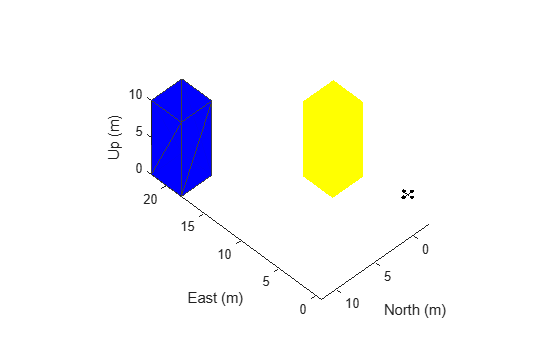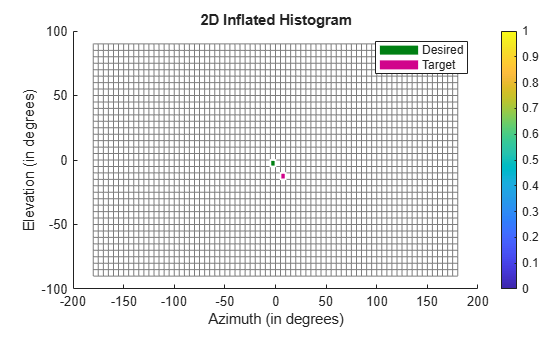# Tune 3D Vector Field Histogram Controller for Obstacle Avoidance in 3D Scene

This example shows how to tune the parameters of the `controllerVFH3D` System object™ to navigate a multirotor UAV in an obstacle environment using a lidar sensor. Use the `uavScenario` object to create the obstacle environment. Mount a lidar sensor on the multirotor UAV to get the positions of obstacles. Use the `uavWaypointFollower` System object to set the goal location for the `controllerVFH3D` object. Use a PID controller to move the UAV in the desired obstacle-free direction.

### Create Obstacle Scene Using UAV Scenario

Remove all variables from the workspace and closes all figures.

```clear close all```

Create a UAV scenario and set the simulation update rate to 2 Hz.

`scene = uavScenario(UpdateRate=2);`

Add polygonal obstacle meshes to the scene.

```addMesh(scene,"polygon",{[8 -2;12 -2;12 2;8 2],[0 10]},0.651*ones(1,3)) addMesh(scene,"polygon",{[18 8;22 8;22 12;18 12],[0 10]},0.651*ones(1,3))```

Visualize the scenario in 3D.

`show3D(scene);`### Create Multirotor Guidance Model and UAV Waypoint Follower

Create the `multirotor` guidance model.

`model = multirotor;`

Create the waypoint follower. Set the UAV type, transition radius for each waypoint, and the waypoints for the UAV to follow.

```wf = uavWaypointFollower(UAVType="multirotor", ... TransitionRadius=1, ... Waypoints=[0 0 -5; 0 20 -5; 20 20 -5]);```

### Create UAV Platform and Mount Sensor

Specify the initial pose of the UAV.

`uavPose = [0 0 -5 pi/2 0 0]';`

Create a quadrotor platform using a north-east-down (NED) reference frame. Specify the initial position and orientation.

```plat = uavPlatform("UAV",scene, ... ReferenceFrame="NED", ... InitialPosition=uavPose(1:3)', ... InitialOrientation=eul2quat(uavPose(4:6)'));```

Add a quadrotor mesh for visualization. Add a rotation to orient the mesh to the UAV body frame.

`updateMesh(plat,"quadrotor",{1.5},[0 0 0],eul2tform([0 0 pi]))`

Create a statistical sensor model to generate point clouds for the lidar sensor.

```lidarmodel = uavLidarPointCloudGenerator(AzimuthResolution=0.3324099, ... ElevationLimits=[-10 30], ... AzimuthLimits=[-60 60], ... ElevationResolution=0.5, ... MaxRange=10, ... UpdateRate=2, ... HasOrganizedOutput=true);```

Create a lidar sensor and mount the sensor on the quadrotor. Specify a mounting location for the sensor that is relative to the UAV body frame.

```lidar = uavSensor("Lidar",plat,lidarmodel, ... MountingLocation=[0 0 -0.4], ... MountingAngles=[0 0 180]);```

### Set Up Simulation and Visualize Scenario

Visualize the scene. Remove edges from the floor mesh.

```figure(1) [ax,plotFrames] = show3D(scene); ax.Children(end).LineStyle = "none";```

Update the plot view for better visibility.

```xlim(ax,[-10 40]) ylim(ax,[-10 40]) zlim(ax,[0 20]) view(ax,2) axis(ax,"equal") hold(ax,"on")```### Configure `ControllerVFH3D` Object and Integrate with Waypoint Follower

Create a `controllerVFH3D` object. Configure the `HistogramResolution` and `MaxAge` properties of the `controllerVFH3D` object to improve the obstacle avoidance. A low `HistogramResolution` value captures most of the obstacle points, but increases computation time. You can use the `MaxAge` property to store obstacle points from previous time steps for computation of a stable obstacle-free direction, and for avoiding local minima. Set the sensor-related parameters.

```vfh3D = controllerVFH3D(HistogramResolution=5, ... MaxAge=0, ... HorizontalSensorFOV=lidarmodel.AzimuthLimits, ... VerticalSensorFOV=lidarmodel.ElevationLimits, ... SensorLocation=lidar.MountingLocation, ... SensorOrientation=lidar.MountingAngles([3 2 1]));```

The navigation logic uses two lookahead distances: one for the waypoint follower, and the other for the `controllerVFH3D` object. The lookahead point for the waypoint follower is the target position for the `controllerVFH3D` object. Compute the desired position for the PID controller from the desired direction output of the `controllerVFH3D` object. A larger lookahead distance for the waypoint follower ensures that the target position is ahead of the desired position. This improves tracking by the PID position controller.

```% Specify lookahead distance for the desired point along obstacle-free % direction, and for the waypoint follower. lookaheadOA = 2; lookaheadWF = 6; % Set up UAV initial state. The UAV state space is position, linear velocity, % attitude Euler angles, angular velocity, thrust and integral error. uavPosition = uavPose(1:3); uavOrientation = eul2quat(uavPose(4:6)')'; intErr = [0;0;0]; initialState = [uavPosition(1); uavPosition(2); uavPosition(3); ... 0; 0; 0; uavPose(4); 0; 0; 0; 0; 0; 0; intErr]; % Set the PID controller parameters. kp = 15; kd = 12; ki = 0.005; kyaw = 10; % Set up simulation start time and integration interval. tStart = 0; tStep = 0.1; % Solution time points. hStep = tStep/10; % Number of iterations. numIter = 200; % Store time and states. tTotal = zeros(numIter*(tStep/hStep+1),1); % Number of states is equal to the number of model states plus % integral error. numStates = numel(model.state)+3; states = zeros(numIter*(tStep/hStep+1),numStates);```

### Tune `ControllerVFH3D` Object

The `controllerVFH3D` object has a number of properties that you must tune to obtain the desired obstacle avoidance behavior.

#### Tune Lidar Sensor Range

Modify the obstacle avoidance algorithm behavior can be modified by changing the range sensor distance limits of the `controllerVFH3D` object.

`vfh3D.DistanceLimits = [0.1 5];`

#### UAV Path Weights

Modify the path of the UAV by changing the weights associated with the target direction, previous direction, and desired direction.

```vfh3D.TargetDirectionWeight = 5; vfh3D.PreviousDirectionWeight = 2; vfh3D.CurrentDirectionWeight = 2;```

Modify the UAV radius and the safety distance properties to control the proximity of the UAV to the obstacles.

```vfh3D.VehicleRadius = 0.5; vfh3D.SafetyDistance = 0.5; ```

### Simulate Obstacle Avoidance in UAV Scenario

Set up the simulation. Then, iterate through the positions and show the scene each time the lidar sensor updates. Advance the scene, move the UAV platform, and update the sensors.

```setup(scene) % Simulate for idx = 1:numIter [isupdated,lidarSampleTime,pt] = read(lidar); xLidar = reshape(pt.Location(:,:,1),[],1); yLidar = reshape(pt.Location(:,:,2),[],1); zLidar = reshape(pt.Location(:,:,3),[],1); sensorPoints = [xLidar yLidar zLidar]; [targetPosition,~,desYaw] = wf(uavPose(1:4),lookaheadWF); [desiredDirection,desiredYaw,status] = vfh3D(uavPosition,uavOrientation, ... sensorPoints,targetPosition); % Visualize the histogram. figure(2) show(vfh3D,PlotsToShow="2D Inflated Histogram"); % Select desired position along the obtacle-free direction. desiredPosition = uavPosition + lookaheadOA*desiredDirection; [t,y] = ode23(@(t,x)exampleHelperDerivative(t,x,model,desiredPosition,desiredYaw,kp,kd,ki,kyaw), ... tStart:hStep:tStart + tStep,initialState); % Store data. tTotal((idx-1)*(tStep/hStep+1) + 1:idx*(tStep/hStep+1)) = t; states((idx-1)*(tStep/hStep+1) + 1:idx*(tStep/hStep+1),:) = y; % Update states. uavPosition = y(end,1:3)'; uavOrientEul = y(end,7:9)'; uavOrientation = eul2quat(uavOrientEul')'; tStart = t(end); initialState = y(end,:); % Plot the path. desPosition = plot3(desiredPosition(2),desiredPosition(1),-desiredPosition(3),".g",Parent=ax); tgtPosition = plot3(targetPosition(2),targetPosition(1),-targetPosition(3),".r",Parent=ax); goalPosition = plot3(wf.Waypoints(end,2),wf.Waypoints(end,1),-wf.Waypoints(end,3),"ob",Parent=ax); legend(ax,[desPosition tgtPosition goalPosition],["Desired Position","Target Position","Goal"]) if isupdated % Use fast update to move platform visualization frames. show3D(scene,Parent=ax,Time=lidarSampleTime,FastUpdate=true); % Move the platform. move(plat,[uavPosition' y(end,4:6) zeros(1,3) eul2quat(uavOrientEul') zeros(1,3)]); drawnow limitrate end % Advance scene simulation time. advance(scene); % Update all sensors in the scene. updateSensors(scene) uavPose = [uavPosition; quat2eul(uavOrientation',"ZYX")']; end```### Visualize Path

Visualize the path of the UAV through the environment.

```figure(3) plot3(states(:,2),states(:,1),-states(:,3)) title("UAV Path") xlabel("East (m)") ylabel("North (m)") zlabel("Altitude (m)")```